Holt McDougal Algebra 2 Chapter 5: Quadratic Functions Chapter Exam

Exam Instructions:

Choose your answers to the questions and click 'Next' to see the next set of questions. You can skip questions if you would like and come back to them later with the yellow "Go To First Skipped Question" button. When you have completed the practice exam, a green submit button will appear. Click it to see your results. Good luck!

Answered 0 of 30

Page 1

Question 2 2. Simplify.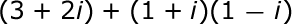Question 5 5. What would the first step be in completing the square of the following equation?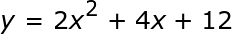Page 2

Question 9 9. Solve the equation below.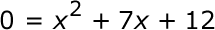Page 3

Question 13 13. What is the simplified value of the expression below?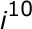Question 14 14. What would be the first step in dividing the complex numbers below?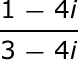Question 15 15. Simplify.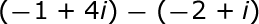Page 4

Question 16 16. Simplify.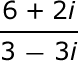Question 17 17. Rewrite the following equation in the vertex form.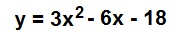Page 5

Question 22 22. Simplify.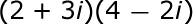Question 23 23. What type of graph is this?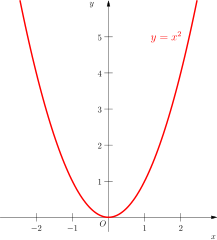Question 24 24. What is the most simplified form of the expression below?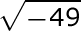Page 6

Question 27 27. Which number is represented by the graph below?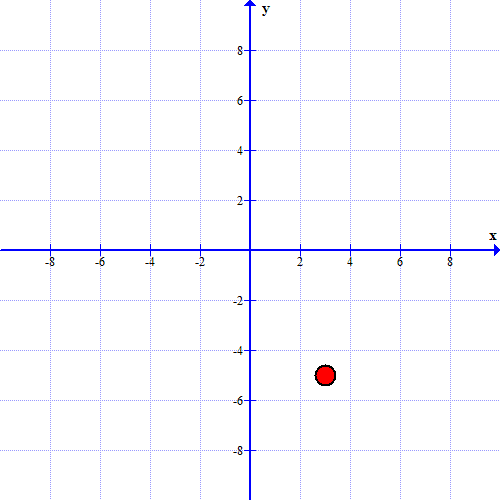Question 28 28. Use the quadratic formula to find the solutions to the equation below.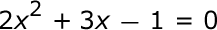Question 29 29. What is the complex conjugate of the expression below?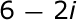Holt McDougal Algebra 2 Chapter 5: Quadratic Functions Chapter Exam Instructions

Choose your answers to the questions and click 'Next' to see the next set of questions. You can skip questions if you would like and come back to them later with the yellow "Go To First Skipped Question" button. When you have completed the practice exam, a green submit button will appear. Click it to see your results. Good luck!

Support